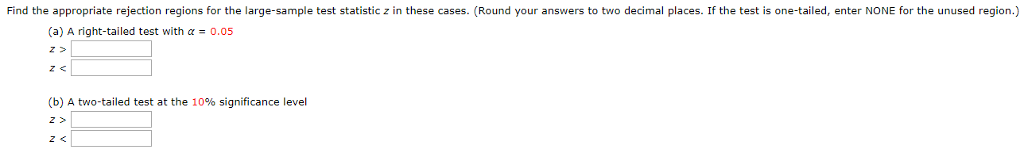# Question Find the appropriate rejection regions for the large-sample test statistic z in these cases. (Round your answers to two decimal places. If the test is one-tailed, enter NONE for the unused region.) (a) A right-tailed test with α-0.05 (b) A two-tailed test at the 10% significance level Z >6TKHEF The Asker · Probability and StatisticsTranscribed Image Text: Find the appropriate rejection regions for the large-sample test statistic z in these cases. (Round your answers to two decimal places. If the test is one-tailed, enter NONE for the unused region.) (a) A right-tailed test with α-0.05 (b) A two-tailed test at the 10% significance level Z >
More
Transcribed Image Text: Find the appropriate rejection regions for the large-sample test statistic z in these cases. (Round your answers to two decimal places. If the test is one-tailed, enter NONE for the unused region.) (a) A right-tailed test with α-0.05 (b) A two-tailed test at the 10% significance level Z >